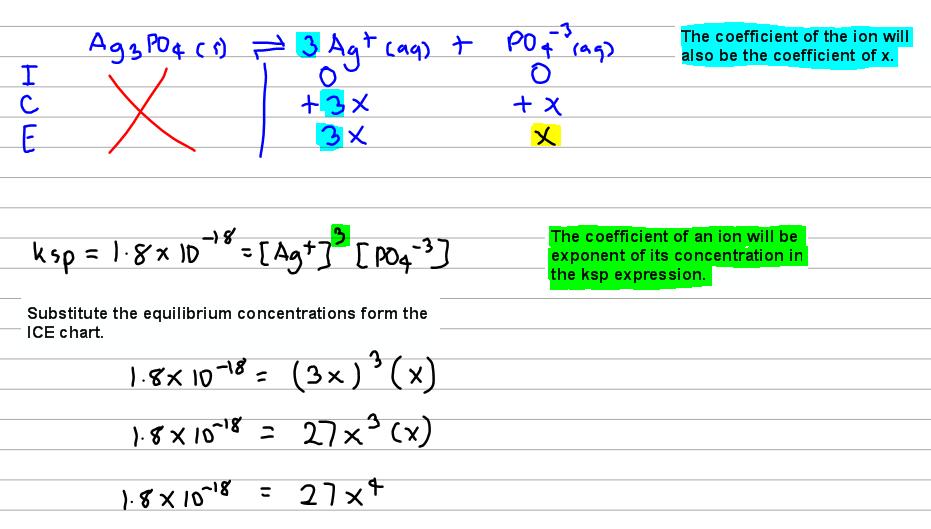# Problem: The Ksp for Ag3PO4 is 1.8 x 10-18. Calculate the molar solubility of Ag 3PO4.

93% (85 ratings)
###### FREE Expert Solution93% (85 ratings)###### Problem Details

The Ksp for Ag3PO4 is 1.8 x 10-18. Calculate the molar solubility of Ag 3PO4.

What scientific concept do you need to know in order to solve this problem?

Our tutors have indicated that to solve this problem you will need to apply the Ksp concept. You can view video lessons to learn Ksp. Or if you need more Ksp practice, you can also practice Ksp practice problems.

What is the difficulty of this problem?

Our tutors rated the difficulty ofThe Ksp for Ag3PO4 is 1.8 x 10-18. Calculate the molar solub...as medium difficulty.

How long does this problem take to solve?

Our expert Chemistry tutor, Dasha took 5 minutes and 8 seconds to solve this problem. You can follow their steps in the video explanation above.

What professor is this problem relevant for?

Based on our data, we think this problem is relevant for Professor Cruz's class at USF.